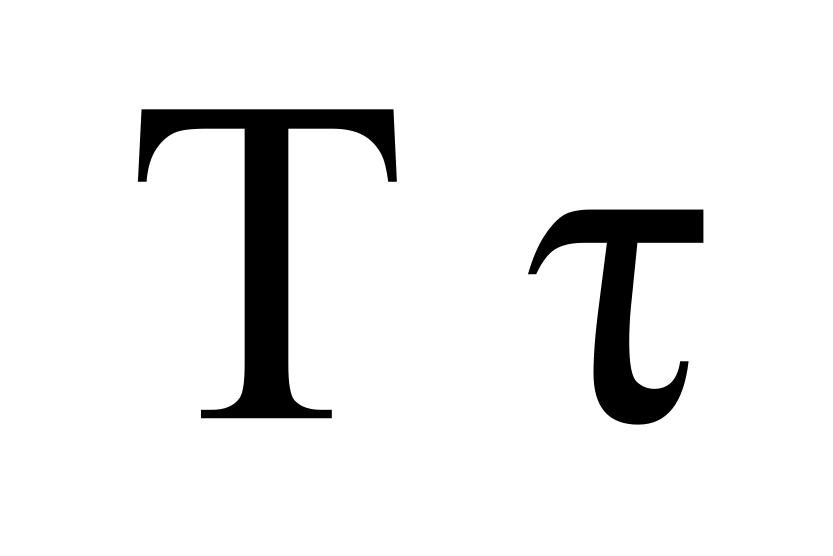* indicates a required field• phone number format invalid• email format invalidProblems detected, please review the form.
Privacy - Terms

# News from Mathnasium of Avon

### Tau vs. Pi

Jun 26, 2019Remember Pi Day, back on 3/14?

Well, pi has a friend called tau, and 6/28 is its day. And at Mathnasium math learning center we are all about celebrating mathematical constants. And variables. And anything that has to do with kids learning math. This one’s for the high school math students.

There’s a whole discussion/debate on the internet about the relative merits of tau and pi and why one is better than the other. See tauday.org to get started. There’s a “manifesto.” There are TED Talks. You can go there, watch that, buy the T-shirt. Since it's almost Tau Day, we're here to describe the virtues of tau.

What’s all the excitement? you ask.

Tau is just 2 times pi.

τ = 2 π

So what’s the big deal? Why do we even need tau, then?

Well obviously we don’t, since you’ve never even heard of it and you are a successful math student. You’ve even done trigonometry!

Oh, wait, what was that? You find trigonometry a little difficult?

Well, yes, it can be a little complicated converting between degrees and radians. You have to remember that π radians is 180 degrees and so 2π radians is a full circle and π/2 radians is 90 degrees.

Everyone knows 360 degrees is a full circle and 90 degrees is a right angle. You’ve probably known that for a long time.

And you know what it means to do a 180. It means turn around so you’re pointed back the way you came from: 180 degrees makes a straight line.

But how many radians makes a straight line? How many radians makes a full circle?

In trigonometry a lot of calculations are done using radians rather than degrees. Instead of finding the sine of 90 degrees or the cosine of 180, you’re asked to find the tangent of π radians or the cotangent of π/4. Now you need to either remember how many degrees that is or ask your calculator to take care of the conversion for you, and then find the solution.

This is where tau (τ) comes in.

Because τ radians is 360 degrees, i.e. a full-circle angle, when you see “τ/4 radians” you immediately know that is a quarter circle. Half of tau — τ/2 — radians is a half circle. 3τ radians is three full circles.

Now let’s talk about using pi and tau in circle geometry.

The familiar formula for area is A = π r2.

The familiar formula for circumference is C = 2 π r.

π is how many diameters of a circle are in its circumference. This would be a good constant to use if we had our equations in terms of d (diameter) rather than r.

But τ is how many radii of a circle are in its circumference, and it fits better with equations in terms of radius.

C = τ r

and A = ½ τ r2

The circumference equation clearer just got simpler, but wait, you say, the area equation got more complicated!

Or maybe not.

If you’ve taken a physics class you might have seen these equations:

The distance an object has fallen due to gravity:  y = ½gt2

The kinetic energy of an object:  K = ½mv2

The potential energy in a spring:  U = ½kx2

Suddenly A = ½τr2 seems like an easy enough formula to remember.

Anyway, area is nice but circumference is where the circle is really at.

The circle itself is the curved line whose measure we call “circumference.” In everyday-speak, like at the arts and crafts table where you’re trying to draw smiley faces, a “circle” might be a disc, a thing that has area. A Frisbee or a CD or a perfectly shaped sugar cookie could be called a circle. But in mathematical terms a circle is not its area. The area inside a circle is, well, the area inside a circle.

To recap:

The ratio of the circumference of a circle to its radius (i.e. tau) is interesting and important because radius is essential to defining the circle (the set of all points equidistant from a given point in a plane), and that’s where the notion of radians comes from. When we work in units of radians, it can be helpful to use tau is a reference constant.

Happy tau day!